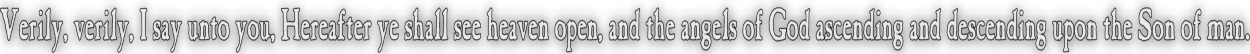None:
Polyps:
Strongs:CreationismGod And Subgroup Addition In the metamath and revelation sections It was made plain that there is an addition on K4 subgroups of the octal group equal to the complement of their symmetric difference in the octal. i.e. (A v B)c I.e; (0,a,b,c) + (0,a,d,e) = (0,a,b,c,d,e,f,g) - {b,c,d,e) = (0,a,f,g). There has been a distinction thus far between the "earth" elements that are the cosets of a given K4 subgroup, so that if using the multiplication of (a,b,d,c,f,g,e) which holds the triples {b,d,f} and {c,e,g} static, not only does multiplication preserve the subgroups, likewise the cosets in "earthy elements" are also preserved. So if (a,b,c) is sent to (b,d,f), then {d,e,f,g} is sent to {a,c,e,g} etc; We also noted that in GF(8) the frobenius map will always hold fixed a static subgroup. Likewise it holds fixed the triple of elements in the group's coset that are not unity. It is structurally impossible in the octal for the fixed unity to be inside the static subgroup (or indeed the static triple in the coset): we would need to change to the K4 form of the trinity to have that. There are but a few things to note at this stage; That the octal itself is an adequate identity element for K4 subgroup addition. Ie. (0,a,b,c,d,e,f,g) + (0,a,b,c) = (0,a,b,c,d,e,f,g) - (d,e,f,g) = (0,a,b,c) We will refer to static triples as "bows" and static subgroups only as static subgroups. There will be use of Demorgans Laws for 'meet and join' operations. These laws state (Ac ∪ Bc) = (A ∩ B)c and (Ac ∩ Bc) = (A ∪ B)c We will also make particular note of the asociation beteen the sets of "earthy" elements in the dialectic bows of the first four seals of revelation. Each of the four elements say {d,e,f,g} is obviously the complement of the other three when restricted to that set of four, yet we will assert an equivalence between them and their complements. For now we will assume a map T from a set to it's complement. Then, T(A ∩ B) = (T(A) ∪ T(B)) and T(A ∪ B) = (T(A) ∩ T(B)) And T(d) simply maps 'd' to {e,f,g} etc We should note that two cosets under the same addition of K4 subgroups produce strange results. {d,e,f,g} + {a,c,e,g} = (0,a,b,c,d,e,f,g) - {a,c,d,f} = (0,b,e,g) which is a subgroup and is the only other possible subgroup in the octal containing "b", (As the cosets were of (a,b,c) and (b,d,f).) Continue To Next Page Return To Section Start'